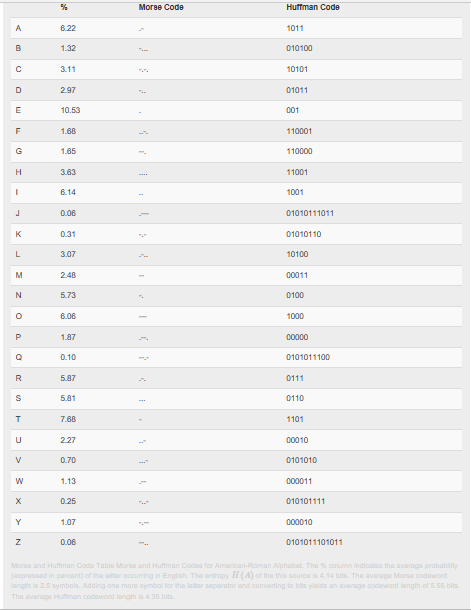6.23: Subtlies of Coding

Learning Objectives

• Some subtleties of coding, including self synchronization and a comparison of the Huffman and Morse codes.

In the Huffman code, the bit sequences that represent individual symbols can have differing lengths so the bitstream index m does not increase in lock step with the symbol-valued signal's index n. To capture how often bits must be transmitted to keep up with the source's production of symbols, we can only compute averages. If our source code averages $\overline{B(A)}$ bits/symbol and symbols are produced at a rate R, the average bit rate equals

$\overline{B(A)}R$

and this quantity determines the bit interval duration

Exercise $$\PageIndex{1}$$

Calculate the relation between T and the average bit rate $\overline{B(A)}R$

$T=\frac{1}{\overline{B(A)}R}$

A subtlety of source coding is whether we need "commas" in the bitstream. When we use an unequal number of bits to represent symbols, how does the receiver determine when symbols begin and end? If you created a source code that required a separation marker in the bitstream between symbols, it would be very inefficient since you are essentially requiring an extra symbol in the transmission stream.

Note

A good example of this need is the Morse Code: Between each letter, the telegrapher needs to insert a pause to inform the receiver when letter boundaries occur.

As shown in this example, no commas are placed in the bitstream, but you can unambiguously decode the sequence of symbols from the bitstream. Huffman showed that his (maximally efficient) code had the prefix property: No code for a symbol began another symbol's code. Once you have the prefix property, the bitstream is partially self-synchronizing: Once the receiver knows where the bitstream starts, we can assign a unique and correct symbol sequence to the bitstream.

Exercise $$\PageIndex{1}$$

Sketch an argument that prefix coding, whether derived from a Huffman code or not, will provide unique decoding when an unequal number of bits/symbol are used in the code.

Solution

Because no codeword begins with another's codeword, the first codeword encountered in a bit stream must be the right one. Note that we must start at the beginning of the bit stream; jumping into the middle does not guarantee perfect decoding. The end of one codeword and the beginning of another could be a codeword, and we would get lost.

However, having a prefix code does not guarantee total synchronization: After hopping into the middle of a bitstream, can we always find the correct symbol boundaries? The self-synchronization issue does mitigate the use of efficient source coding algorithms.

Exercise $$\PageIndex{1}$$

Show by example that a bitstream produced by a Huffman code is not necessarily self-synchronizing. Are fixed-length codes self synchronizing?

Solution

Consider the bitstream …0110111… taken from the bitstream 0|10|110|110|111|…. We would decode the initial part incorrectly, then would synchronize. If we had a fixed-length code (say 00,01,10,11), the situation is much worse. Jumping into the middle leads to no synchronization at all!

Another issue is bit errors induced by the digital channel; if they occur (and they will), synchronization can easily be lost even if the receiver started "in synch" with the source. Despite the small probabilities of error offered by good signal set design and the matched filter, an infrequent error can devastate the ability to translate a bitstream into a symbolic signal. We need ways of reducing reception errors without demanding that pe be smaller.

Example $$\PageIndex{1}$$:

The first electrical communications system—the telegraph—was digital. When first deployed in 1844, it communicated text over wireline connections using a binary code—the Morse code—to represent individual letters. To send a message from one place to another, telegraph operators would tap the message using a telegraph key to another operator, who would relay the message on to the next operator, presumably getting the message closer to its destination. In short, the telegraph relied on a network not unlike the basics of modern computer networks. To say it presaged modern communications would be an understatement. It was also far ahead of some needed technologies, namely the Source Coding Theorem. The Morse code, shown in the table below, was not a prefix code. To separate codes for each letter, Morse code required that a space—a pause—be inserted between each letter. In information theory, that space counts as another code letter, which means that the Morse code encoded text with a three-letter source code: dots, dashes and space. The resulting source code is not within a bit of entropy, and is grossly inefficient (about 25%). The table shows a Huffman code for English text, which as we know is efficient.Contributor

• ContribEEOpenStax